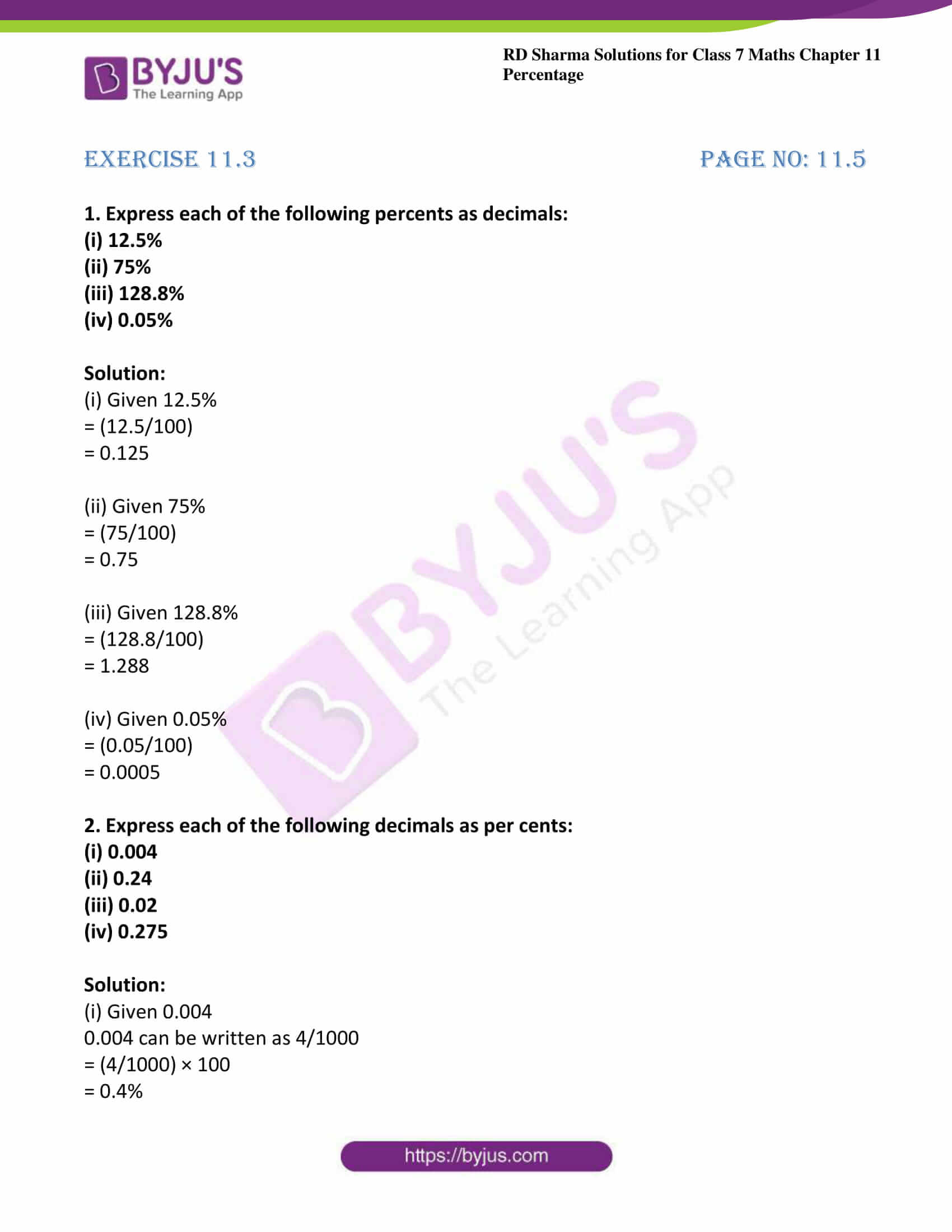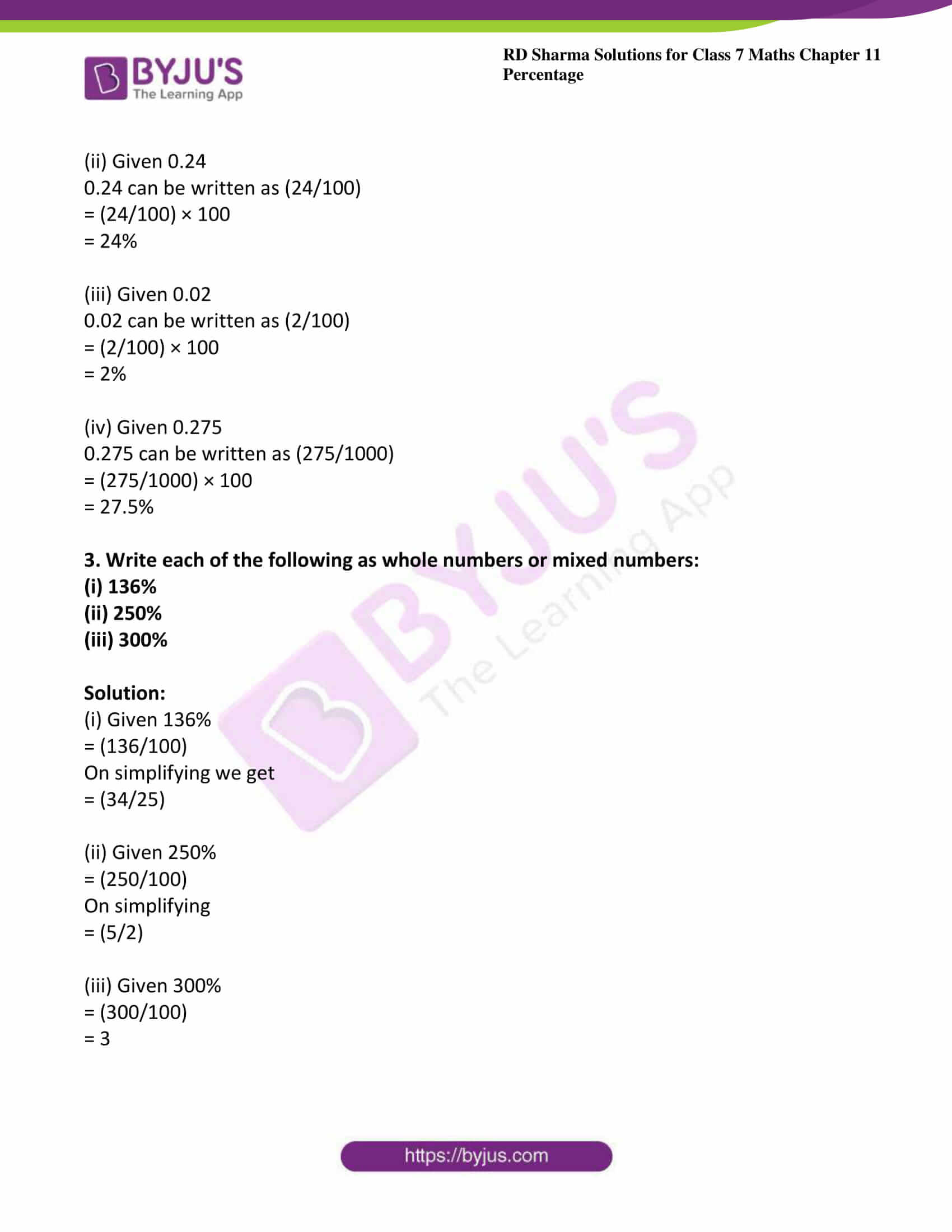# RD Sharma Solutions For Class 7 Maths Chapter 11 Percentage Exercise 11.3

The exercise-wise solutions are depicted in an interactive manner to help students with their exam preparation. The expert faculty at BYJU’S provides students with PDF of solutions to solve the problems in accordance with the CBSE syllabus. RD Sharma Solutions for Class 7 Maths Chapter 11 Percentage Exercise 11.3 are provided here. This exercise gives explanations and a step by step method to convert the given percent into decimal and vice versa. To know more about these topics, click the link provided here of RD Sharma Solutions for Class 7.

## Download the PDF of RD Sharma Solutions For Class 7 Maths Chapter 11 – Percentage Exercise 11.3### Access answers to Maths RD Sharma Solutions For Class 7 Chapter 11 – Percentage Exercise 11.3

Exercise 11.3 Page No: 11.5

1. Express each of the following percents as decimals:

(i) 12.5%

(ii) 75%

(iii) 128.8%

(iv) 0.05%

Solution:

(i) Given 12.5%

= (12.5/100)

= 0.125

(ii) Given 75%

= (75/100)

= 0.75

(iii) Given 128.8%

= (128.8/100)

= 1.288

(iv) Given 0.05%

= (0.05/100)

= 0.0005

2. Express each of the following decimals as per cents:

(i) 0.004

(ii) 0.24

(iii) 0.02

(iv) 0.275

Solution:

(i) Given 0.004

0.004 can be written as 4/1000

= (4/1000) × 100

= 0.4%

(ii) Given 0.24

0.24 can be written as (24/100)

= (24/100) × 100

= 24%

(iii) Given 0.02

0.02 can be written as (2/100)

= (2/100) × 100

= 2%

(iv) Given 0.275

0.275 can be written as (275/1000)

= (275/1000) × 100

= 27.5%

3. Write each of the following as whole numbers or mixed numbers:

(i) 136%

(ii) 250%

(iii) 300%

Solution:

(i) Given 136%

= (136/100)

On simplifying we get

= (34/25)

(ii) Given 250%

= (250/100)

On simplifying

= (5/2)

(iii) Given 300%

= (300/100)

= 3